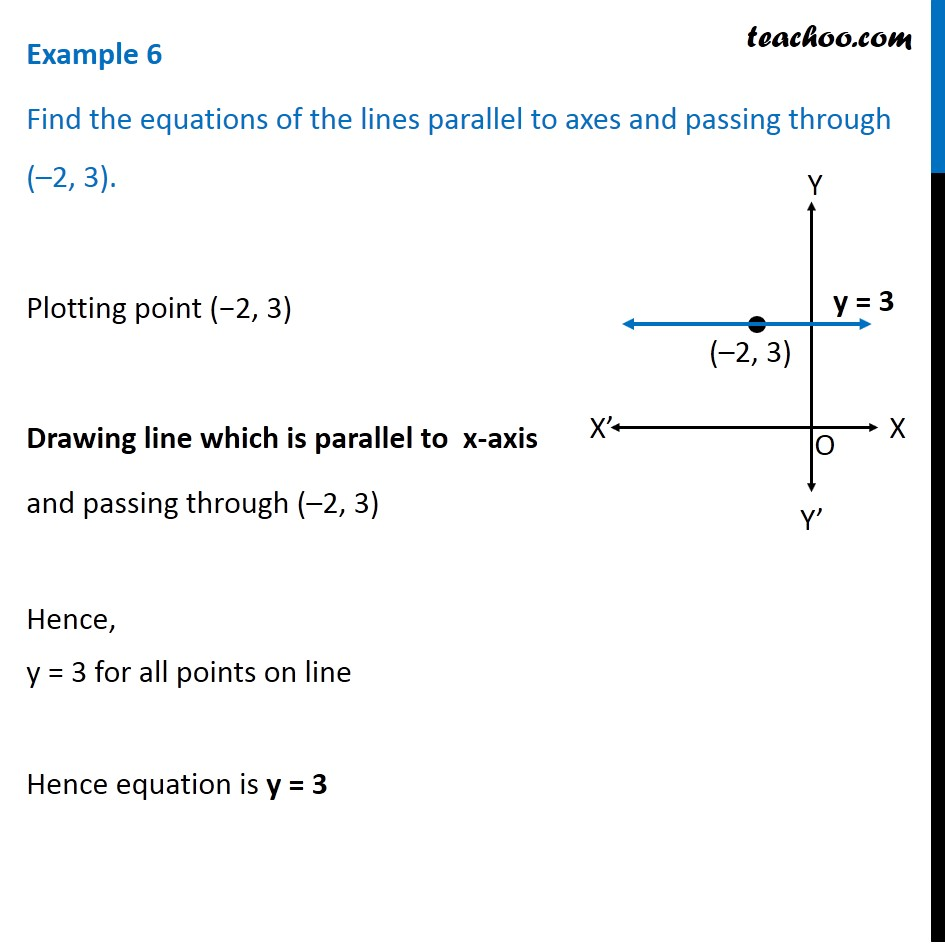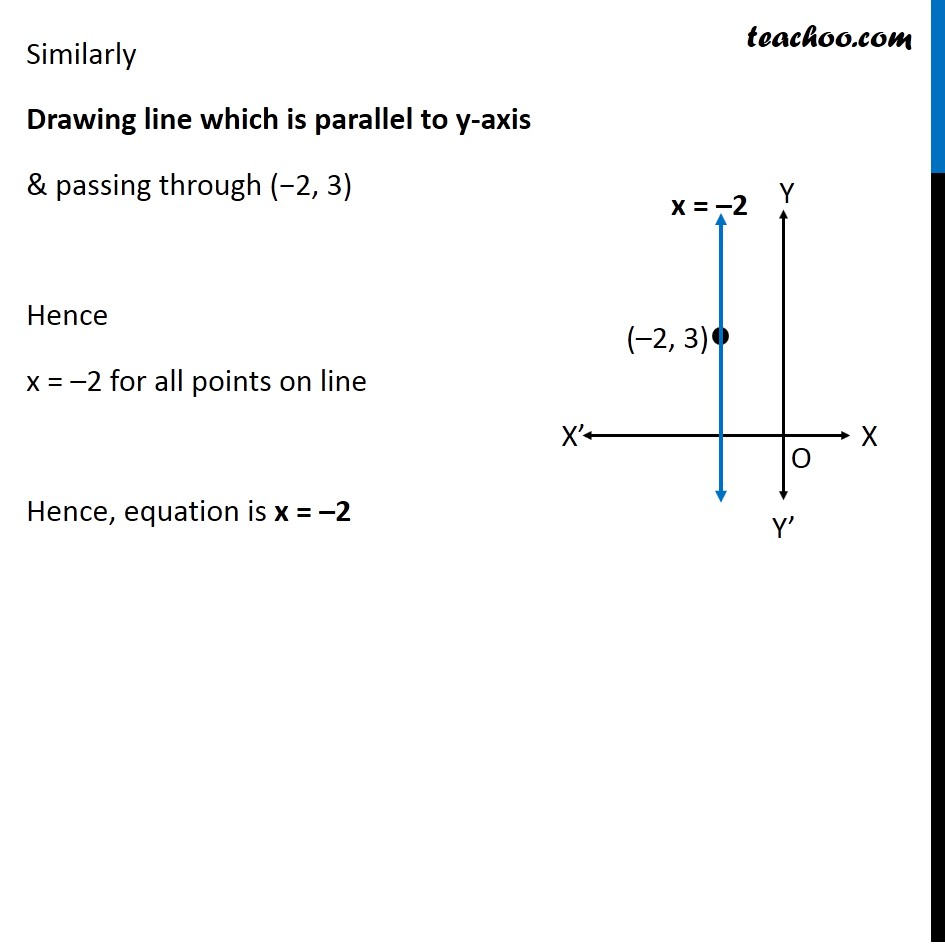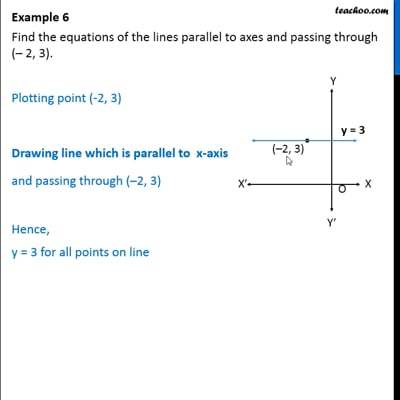Examples

Chapter 10 Class 11 Straight Lines (Term 1)
Serial order wiseThis video is only available for Teachoo black users

### Transcript

Example 6 Find the equations of the lines parallel to axes and passing through (–2, 3). Plotting point (−2, 3) Drawing line which is parallel to x-axis and passing through (–2, 3) Hence, y = 3 for all points on line Hence equation is y = 3 Similarly Drawing line which is parallel to y-axis & passing through (−2, 3) Hence x = –2 for all points on line Hence, equation is x = –2